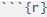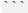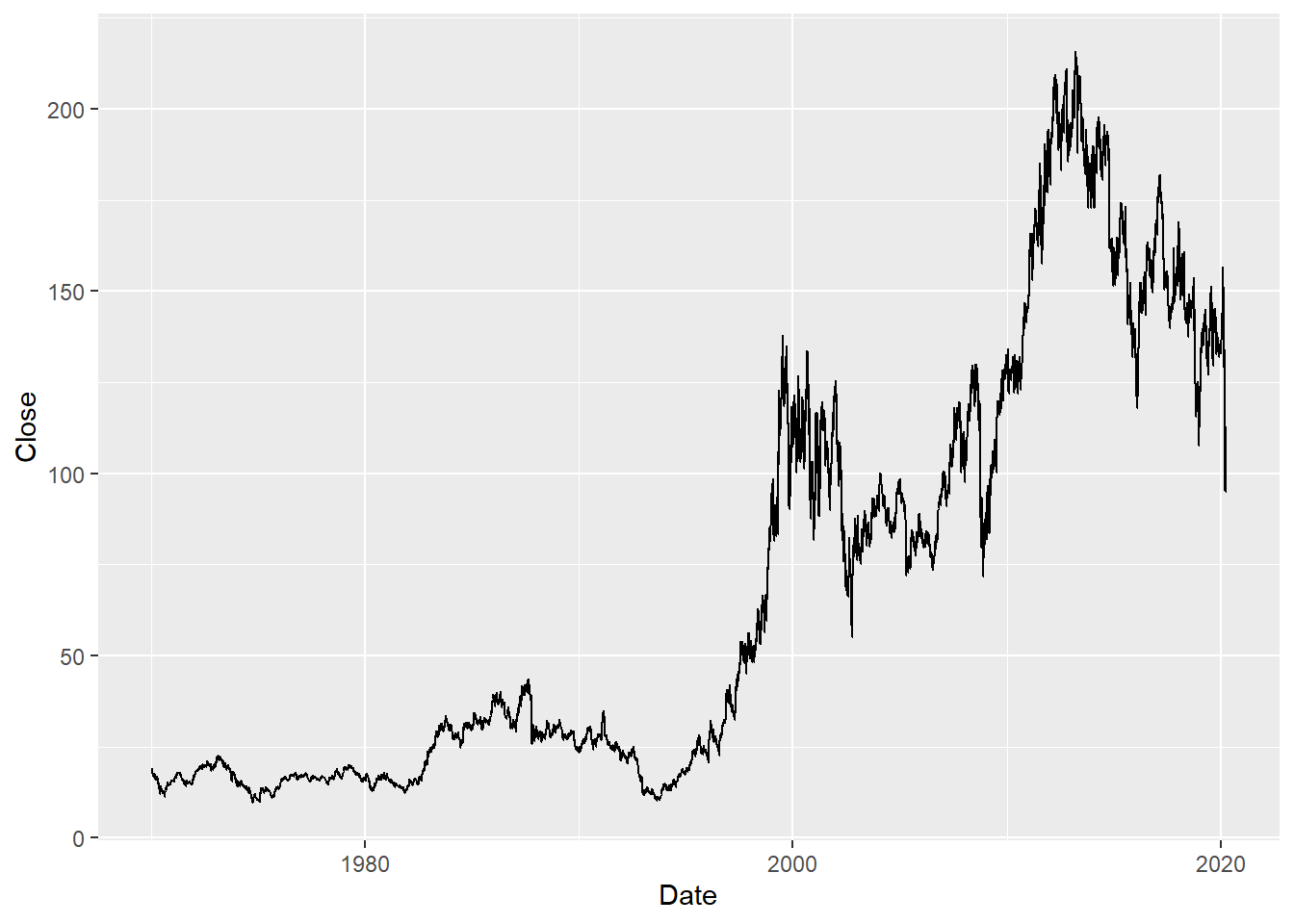# Lab 1: Introduction to R

### Learning objectives:

• setting a working directory (use R Studio projects)
• understanding RMarkdown
• loading R packages (library() function)
• loading data (read_csv() function)
• data frames and variables
• using a variable from a data frame (the $ operator) • plotting a line graph • renaming a variable • specifying data types with col_types • loading data from your working directory (your project directory) ### Background reading • Chapters 9, 10 and 11 in R for Data Science ### 1. Setting a working directory Let’s start a new project. We can call it “ba” for business analytics. Click on “Project” icon in the upper right corner of R Studio. Select ‘New Project’ a ‘New directory’ choose a folder on your computer where you would like to keep your file for this class. ### 2. RMarkdown Let’s open a new R Markdown document. R Markdown combines text and R code. R code is enclosed inandmarks. For example, if we want R to calculate 2+2 we can type the following: 2+2 ##  4 To execute the R code (rather than knit the entire R Markdown document), we put the cursor on the line we want to execute and either click run, or hold ‘control’ key and press ‘enter’. ### 3. Loading packages Almost anything you do in R requires a package - additional functions. Before we can use any packages we have to ‘load’ them into our R session. We do that by calling the library() function with the name of the package as the only argument. Any package that we want to load needs to be already installed. We need to install a package only once but load it each time we start a new R session. To load in our tidyverse package we type the following: library(tidyverse) ## -- Attaching packages ------------------------------------------------------------------ tidyverse 1.2.1 -- ## v ggplot2 3.2.1 v purrr 0.3.2 ## v tibble 2.1.3 v dplyr 0.8.3 ## v tidyr 1.0.0 v stringr 1.4.0 ## v readr 1.3.1 v forcats 0.4.0 ## -- Conflicts --------------------------------------------------------------------- tidyverse_conflicts() -- ## x dplyr::filter() masks stats::filter() ## x dplyr::lag() masks stats::lag() ### 4. Loading data At last, let’s load some data. We will get data on IBM’s historical stock prices from Yahoo! Finance. (There are other options such as Quandl, but they are not up to date.) I obtained the url below by searching for a ticker (IBM), clicking on ‘Historical Data’, selecting ‘max’ under ‘Time Period’, clicking ‘Apply’, and right-clicking on ‘Download Data’, and selecting ‘Copy Link Address’. #the quanld WIKI ends in 2018 #mydata <- read_csv("https://www.quandl.com/api/v3/datasets/WIKI/AAPL.csv") #we may need to adjust the url for the yahoo finance data mydata <- read_csv("https://query1.finance.yahoo.com/v7/finance/download/IBM?period1=1&period2=2000000000&interval=1d&events=history") ## Parsed with column specification: ## cols( ## Date = col_date(format = ""), ## Open = col_double(), ## High = col_double(), ## Low = col_double(), ## Close = col_double(), ## Adj Close = col_double(), ## Volume = col_double() ## ) Function read_csv() reads comma separated files. It takes the location of a file as an argument. The location can be a path on your hard drive or a URL. In this case the location was a URL. The <- operator assigns the result of the read_csv() function to an object we named ‘mydata’. The result of the read_csv() function is an object called tibble which is a type of data frame. You can examine this data frame by clicking on its name in the Environment window. ### 4. Data frames and variables Data frame (or its type tibble) is an object that holds variables and observations. Let’s examine the structure of our data frame ‘mydata’. We can do this by executing glimpse() function. (We can do this by either entering the function into the console, or if we want it part of the markdown document, entering it and executing it within the markdown document.) glimpse(mydata) ## Observations: 12,675 ## Variables: 7 ##$ Date        <date> 1970-01-02, 1970-01-05, 1970-01-06, 1970-01-07, 1...
## $Open <dbl> 18.22500, 18.30000, 18.41250, 18.42500, 18.43750, ... ##$ High        <dbl> 18.28750, 18.41250, 18.45000, 18.43750, 18.47500, ...
## $Low <dbl> 18.2000, 18.3000, 18.3125, 18.3125, 18.3750, 18.42... ##$ Close       <dbl> 18.23750, 18.41250, 18.42500, 18.43750, 18.47500, ...
## $Adj Close <dbl> 1.515268, 1.529808, 1.530848, 1.531886, 1.535001, ... ##$ Volume      <dbl> 315200, 424000, 488000, 457600, 707200, 585600, 37...

The results tell us that we have almost 12 thousand observations and 7 variables. It tells us the variable names, their type and the first few observations. We see that variable Date is a <date>, and the rest of the variables are <dbl> which means that they are numbers stored with a ‘double’ precision.

### 5. Using a variable from a data frame

If we want to do something with a particular variable we write the name of the data frame, a ‘$’ sign, and the name of the variable. For example, below we calculate the summary of the variable Close in data frame mydata: summary(mydata$Close)
##    Min. 1st Qu.  Median    Mean 3rd Qu.    Max.
##    9.50   18.22   33.00   67.99  113.80  215.80

### IN-CLASS EXERCISE

1. Load in data on the results of the 2016 NHL season from https://dvorakt.github.io/business_analytics/lab1/NHLseason2016.csv
2. How many games were there?
3. What was the average attendance?
4. Did home teams score on average more goals than visiting teams?
nhl <- read_csv("https://dvorakt.github.io/business_analytics/lab1/NHLseason2016.csv")
## Parsed with column specification:
## cols(
##   Date = col_date(format = ""),
##   Visitor = col_character(),
##   Home = col_character(),
##   goals_home = col_double(),
##   goals_visit = col_double(),
##   length = col_time(format = ""),
##   attendance = col_double(),
##   note = col_character()
## )
summary(nhl$attendance) ## Min. 1st Qu. Median Mean 3rd Qu. Max. ## 9021 16440 18192 17572 19070 67246 ### 6. Plotting data Let’s plot the closing price of Netflix. We use a powerful function called ggplot(). The function creates a plot by combining a few components. First, it needs to know the data frame from which to get the data. Second, it needs to know which variables should be on the x and y axes. This is specified in aesthetics(). Finally, it needs to know the geometric object (geom) we want to use to represent the data (in this case a line). ggplot(data=mydata, aes(x=Date, y=Close)) + geom_line()### 7. Renaming variables Some of the variable in our data frame have inconvenient names (e.g. Adj Close has spaces in its name necessitating the use of quotes). We can rename the variable using the function rename() which takes as its first argument a data frame, and as remaining arguments new variable names set equal to old variable names. The function returns a data frame. Below we overwrite our mydata data frame with a new one that includes renamed variable. mydata <- rename(mydata, adj_close=Adj Close) ### 8. Changing data type Let’s load the stock price data for Apple (AAPL). aapl <- read_csv("https://query1.finance.yahoo.com/v7/finance/download/AAPL?period1=1&period2=2000000000&interval=1d&events=history") ## Parsed with column specification: ## cols( ## Date = col_date(format = ""), ## Open = col_character(), ## High = col_character(), ## Low = col_character(), ## Close = col_character(), ## Adj Close = col_character(), ## Volume = col_character() ## ) We see that many of the columns were read as characters rather than numbers. Looking through the raw data I see that there is one row that has characters for the otherwise numerical columns. This causes R to guess that those columns are character columns. Let’s force R to read these columns as numbers. aapl <- read_csv("https://query1.finance.yahoo.com/v7/finance/download/AAPL?period1=1&period2=2000000000&interval=1d&events=history", col_types=cols(Date=col_date(), Open=col_double(), High=col_double(), Low=col_double(), Close=col_double(), Adj Close=col_double(), Volume=col_double())) ## Warning: 6 parsing failures. ## row col expected actual file ## 166 Open a double null 'https://query1.finance.yahoo.com/v7/finance/download/AAPL?period1=1&period2=2000000000&interval=1d&events=history' ## 166 High a double null 'https://query1.finance.yahoo.com/v7/finance/download/AAPL?period1=1&period2=2000000000&interval=1d&events=history' ## 166 Low a double null 'https://query1.finance.yahoo.com/v7/finance/download/AAPL?period1=1&period2=2000000000&interval=1d&events=history' ## 166 Close a double null 'https://query1.finance.yahoo.com/v7/finance/download/AAPL?period1=1&period2=2000000000&interval=1d&events=history' ## 166 Adj Close a double null 'https://query1.finance.yahoo.com/v7/finance/download/AAPL?period1=1&period2=2000000000&interval=1d&events=history' ## ... ......... ........ ...... ................................................................................................................... ## See problems(...) for more details. glimpse(aapl) ## Observations: 9,909 ## Variables: 7 ##$ Date        <date> 1980-12-12, 1980-12-15, 1980-12-16, 1980-12-17, 1...
## $Open <dbl> 0.513393, 0.488839, 0.453125, 0.462054, 0.475446, ... ##$ High        <dbl> 0.515625, 0.488839, 0.453125, 0.464286, 0.477679, ...
## $Low <dbl> 0.513393, 0.486607, 0.450893, 0.462054, 0.475446, ... ##$ Close       <dbl> 0.513393, 0.486607, 0.450893, 0.462054, 0.475446, ...
## $Adj Close <dbl> 0.406782, 0.385558, 0.357260, 0.366103, 0.376715, ... ##$ Volume      <dbl> 117258400, 43971200, 26432000, 21610400, 18362400,...

The function gives us a warning that in row 166 it encountered a character while expecting a number. The values in that row were replaced with NA.

If we wanted to load only two columns, the two were are really interested in, we could use cols_only.

aapl <- read_csv("https://query1.finance.yahoo.com/v7/finance/download/AAPL?period1=1&period2=2000000000&interval=1d&events=history",
col_types=cols(Date=col_date(),
Adj Close=col_double()))
## Warning: 1 parsing failure.
## row       col expected actual                                                                                                                file
## 166 Adj Close a double   null 'https://query1.finance.yahoo.com/v7/finance/download/AAPL?period1=1&period2=2000000000&interval=1d&events=history'

### 8. Loading data from your working directory (project directory)

Loading data from a url is cool because we don’t have to store the data, and the data is automatically updated. However, when the data is large, it is faster to have the data stored locally. Moreover, sometimes we want to work with ‘static’ (i.e. not constantly updated) data. Therefore, let’s save the Apple stock price into the directory/folder where we created our project, and where this RMarkdown document is saved. The function read_csv() will look for any files in that location.

aapl <- read_csv("AAPL.csv",
col_types=cols_only(Date=col_date(),
Open=col_double()))
## Warning: 1 parsing failure.
## row  col expected actual       file
## 166 Open a double   null 'AAPL.csv'

### IN-CLASS EXERCISE

1. Plot the adjusted closing price of IBM.
2. How is it different from the unadjusted price?

## Exercises

Create a new R Markdown file that does the analysis and answers the questions below. Knit your R Markdown into an html, print it and bring to class. Don’t forget to load tidyverse at the beginning of your code.

1. Download data from Yahoo! Finance on closing stock price of GE. (hint: GE’s ticker is GE)
2. How many observations are available for GE?
3. Rename Adj. Close as ge.
4. Plot the adjusted closing prices GE over time. Use this documentation for ggplot2 to add title to your plot.
5. Use the same documentation to create a new plot that uses logarithmic scale on the y axis.
6. Explain why we see different patterns on the linear versus logarithmic scale plots. (Hint 1: Think about what the vertical distances between points represent on each of the graphs.)
7. What are your overall conclusions from these two plots? In other words, what are the key things that can be learned about the behavior of the GE over this time frame?
8. Take a look at the data sources in this document. Pick one, find a .csv data and load it into R. In about 200 words, describe the data (what is the unit of observation, how many columns, how many rows, why you think the data is interesting).